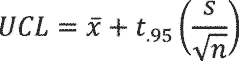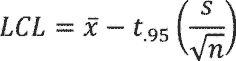# Title 10

You are viewing the current version of the eCFR. The eCFR is up to date as of 7/26/2021.

# Title 10

###### eCFR Content

Editorial codification of the general and permanent rules published in the Federal Register.

§ 429.17 Water heaters.

(a) Determination of represented value.

(1) Manufacturers must determine the represented value for each water heater by applying an AEDM in accordance with 10 CFR 429.70 or by testing for the uniform energy factor, in conjunction with the applicable sampling provisions as follows:

(i) If the represented value is determined through testing, the general requirements of 10 CFR 429.11 are applicable; and

(ii) For each basic model selected for testing, a sample of sufficient size shall be randomly selected and tested to ensure that -

(A) Any represented value of the estimated annual operating cost or other measure of energy consumption of a basic model for which consumers would favor lower values shall be greater than or equal to the higher of:

(1) The mean of the sample, where:and, x is the sample mean; n is the number of samples; and xi is the ith sample;

Or,

(2) The upper 95-percent confidence limit (UCL) of the true mean divided by 1.10, where:And x is the sample mean; s is the sample standard deviation; n is the number of samples; and t0.95 is the t statistic for a 95-percent one-tailed confidence interval with n-1 degrees of freedom (from Appendix A).

(B) Any represented value of the uniform energy factor, or other measure of energy consumption of a basic model for which consumers would favor higher values shall be less than or equal to the lower of:

(1) The mean of the sample, where:and, x is the sample mean; n is the number of samples; and xi is the ith sample;

Or,

(2) The lower 95-percent confidence limit (LCL) of the true mean divided by 0.90, where:And x is the sample mean; s is the sample standard deviation; n is the number of samples; and t0.95 is the t statistic for a 95-percent one-tailed confidence interval with n-1 degrees of freedom (from Appendix A).

(C) Any represented value of the rated storage volume must be equal to the mean of the measured storage volumes of all the units within the sample.

(D) Any represented value of first-hour rating or maximum gallons per minute (GPM) must be equal to the mean of the measured first-hour ratings or measured maximum GPM ratings, respectively, of all the units within the sample.

(b) Certification reports.

(1) The requirements of 10 CFR 429.12 are applicable to water heaters; and

(2) Pursuant to 10 CFR 429.12(b)(13), a certification report shall include the following public, product-specific information:

(i) For storage-type water heater basic models: The uniform energy factor (UEF, rounded to the nearest 0.01), the rated storage volume in gallons (rounded to the nearest 1 gal), the first-hour rating in gallons (gal, rounded to the nearest 1 gal), and the recovery efficiency in percent (%, rounded to the nearest 1%);

(ii) For instantaneous-type water heater basic models: The uniform energy factor (UEF, rounded to the nearest 0.01), the rated storage volume in gallons (gal, rounded to the nearest 1 gal), the maximum gallons per minute (gpm, rounded to the nearest 0.1 gpm), and the recovery efficiency in percent (%, rounded to the nearest 1%); and

(iii) For grid-enabled water heater basic models: The uniform energy factor (UEF, rounded to the nearest 0.01), the rated storage volume in gallons (gal, rounded to the nearest 1 gal), the first-hour rating in gallons (gal, rounded to the nearest 1 gal), the recovery efficiency in percent (%, rounded to the nearest 1%), a declaration that the model is a grid-enabled water heater, whether it is equipped at the point of manufacture with an activation lock, and whether it bears a permanent label applied by the manufacturer that advises purchasers and end-users of the intended and appropriate use of the product.

[81 FR 96235, Dec. 29, 2016]